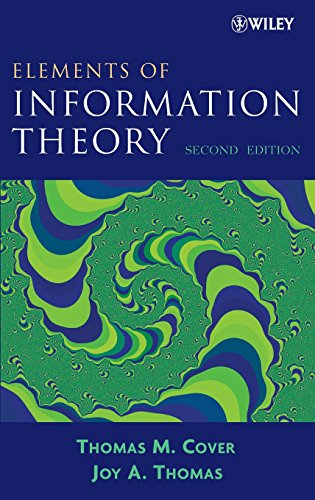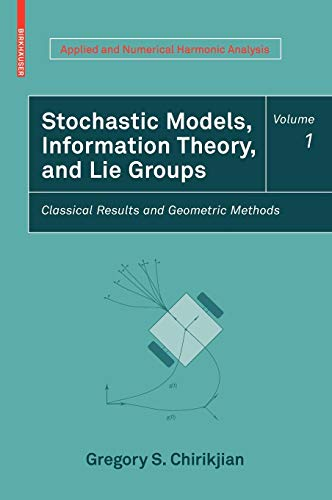# Best Information Theory Books to Learn All About it's Applications

Information Theory is the mathematical study of the coding of information in the form of sequences of symbols, impulses, etc. and of how rapidly such information can be transmitted, for example through computer circuits or telecommunication channels. It was founded by Claude Shannon toward the middle of the twentieth century and has since then evolved into a vigorous branch of mathematics fostering the development of other scientific fields, such as statistics, biology, behavioral science, neuroscience, and statistical mechanics. By reading these books you can learn about the ins and outs of Information Theory.

Here you will get some of the best Information Theory Books

## 1. An Introduction to Information Theory: Symbols, Signals and Noise (Dover Books on Mathematics)

An Introduction to Information Theory is an intelligent and resourceful review of Information Theory. Information theory is the mathematical treatment of the concepts, parameters, and rules governing the transmission of messages through communication systems. Written by John R. Peirce, this book provides a solid understanding of Information Theory. The author begins with the brilliant formulations of Claude Shannon and describes the meaning of such aspects like encoding and binary digits, entropy, language and meaning, efficient encoding, and the noisy channel. He then covers the complex and strict confines of the topic to find a way in which physics, cybernetics, psychology, and art are related to Information Theory. This is a must-read for everyone keen to learn about Information Theory.

Topics included:

• The origins of information theory
• Mathematical model
• Encoding binary digits
• Entropy and Efficient encoding
• The noisy channel
• Information Theory and Physics
• Cybernetics
• Information theory and Psychology and Art and many more

## 2. Information Theory: A Tutorial Introduction

Information Theory: A tutorial Introduction is a very approachable and intuitive introduction to information theory. Written by James V Stone this book has the clearest explanation which is applicable to all and of any mathematical topic to date. Thoughtful explanation and walk-through of the complex topics made this book one of it's kind. This Information Theory book starts out by defining the units of information, bits and then how to quantify information so that it has the properties that we would like it to have namely additive, continuous, maximal with respect to certain probability measures and symmetry. He goes through some of the various examples to give a feel of how information can be considered and how many means of communication include much redundancy. The author then moves on from communication-related information theory to entropy and physics covering all the topics in between.

Topics included:

• Introduction to Information Theory
• Entropy of discrete and continuous variables
• Source coding theorem
• Noisy channel coding theorem
• Mutual information
• Channel capacity
• Thermodynamic entropy and information.

## 3. Elements of Information Theory 2nd Edition (Wiley Series in Telecommunications and Signal Processing)Author: Thomas M. Cover,Joy A. Thomas
Published at: 18/07/2006
ISBN: 0471241954

Elements of Information Theory is a good read for learning Information Theory. Written by two genius Thomas M. Cover and Joy A. Thomas this book has all the essential topics in Information Theory covered in detail. Topics include entropy, data compression, channel capacity, rate-distortion, network information theory, and hypothesis testing. They provide readers with a solid understanding of the underlying theory and applications. Problem sets and a telegraphic summary at the end of each chapter further assist readers. Also, it has a lot of examples to practice. This book has become an established favorite in university courses on information theory. that's why this is the best Information Theory books.

Topics included:

• Entropy and Mutual Information
• Asymptotic Equipartition Property
• Entropy Rates of a Stochastic Process
• Data Compression
• Channel Capacity and Gaussian Channel
• Rate-Distortion Theory
• Information Theory and Statistics
• Universal source coding
• Network information theory and many more.

## 4. Introduction To Black Holes, Information And The String Theory Revolution, An: The Holographic Universe

This book covers the interesting topics and mathematical explanation of Black Holes and both Information and String theory. It is brief and action-packed with formulas and simple explanations, a great balance of theory and narrative which very succinctly covers the topics of black holes and how they are described by string theory. There is no other introductory text that goes as deep into holographic theories and entropy bounds as this one. Furthermore, the book is well-written, contains clear illustrations, and appeals to the reader's intuition.

Chapters include:

• Black holes and quantum mechanics
• The entropy of the free quantum fields
• Thermodynamics of black holes
• The laws of nature
• Entropy bounds
• The holographic principle
• String theory

## 5. Science and Information Theory: Second Edition (Dover Books on Physics)

Science and Information theory is a collaboration of the relation between Information Theory and Physics. As one of the pioneers of information theory based on entropy and/or negentropy, the author presented the concepts and related topics with deep insights and exceptionally solid logic in this book. The author applies the principles of information theory to a variety of issues, including Maxwell's demon, thermodynamics, and measurement problems. The author also applies the principles of coding, coding problems and solutions, the analysis of signals, a summary of thermodynamics, thermal agitation, and Brownian motion, and thermal noise in an electric circuit. The later chapters also discuss the associations between information theory, the uncertainty principle, and physical limits of observation, in addition to problems related to computing, organizing information, and inevitable errors.

Chapters include:

• Definition of Information Theory
• Principle of coding
• The capacity of a channel discussion
• Coding problems and error handling
• Signal analysis
• The Negentropy principle of Information
• Information theory and the uncertainty principle
• Information, Organization and other Problems

## 6. Stochastic Models, Information Theory, and Lie Groups, Volume 1: Classical Results and Geometric Methods (Applied and Numerical Harmonic Analysis)Author: Gregory S. Chirikjian
Published at: 15/09/2009
ISBN: 081764802X

This book is based on a deep understanding of Stochastic Models, Information Theory, and Lie Groups. This book achieves a transparent and deep illustration of the mathematics of complex stochastic modeling for well-motivated engineering students. This book is well recommended for undergraduate and graduate students, researchers and those who are working in applied mathematics. This book has extensive exercises and motivating examples that will make the work suitable as a textbook for use in courses that emphasize applied stochastic processes or differential geometry.

Contents include:

• Modern Mathematical Notation and Terminology
• Gaussian distribution and the heat equation
• Probability and Information Theory
• Stochastic differential equation
• The geometry of curves and surfaces
• Polytopes and manifolds

## 7. Quantum Information Theory

Quantum Information Theory is an ideal option for graduate students to learn Quantum Information Theory. This book explains Quantum Mechanics with Quantum Information Theory. It also offers careful studies of the important protocols of teleportation, superdense coding, and entanglement distribution. In this revised and new edition of this book readers can expect to find over 100 pages of new material, including detailed discussions of Bell's theorem, the CHSH game, Tsirelson's theorem, the axiomatic approach to quantum channels, the definition of the diamond norm and its interpretation, and a proof of the Choi-Kraus theorem. Also, discussion of the importance of the quantum dynamic capacity formula has been completely revised, and many new exercises and references have been added.

Chapters include:

• Concepts of information and quantum theory
• Noiseless and noisy quantum theory
• Different kinds of quantum protocols
• Tools of Quantum Shannon Theory
• Quantum Information and Entropy
• Classical and Quantum Typicality
• Deep analysis of noisy and noiseless Quantum Information Theory

Thanks for reading this post. If you have any opinion don't hesitate to comment here. Also please subscribe our newsletter to get more updates.